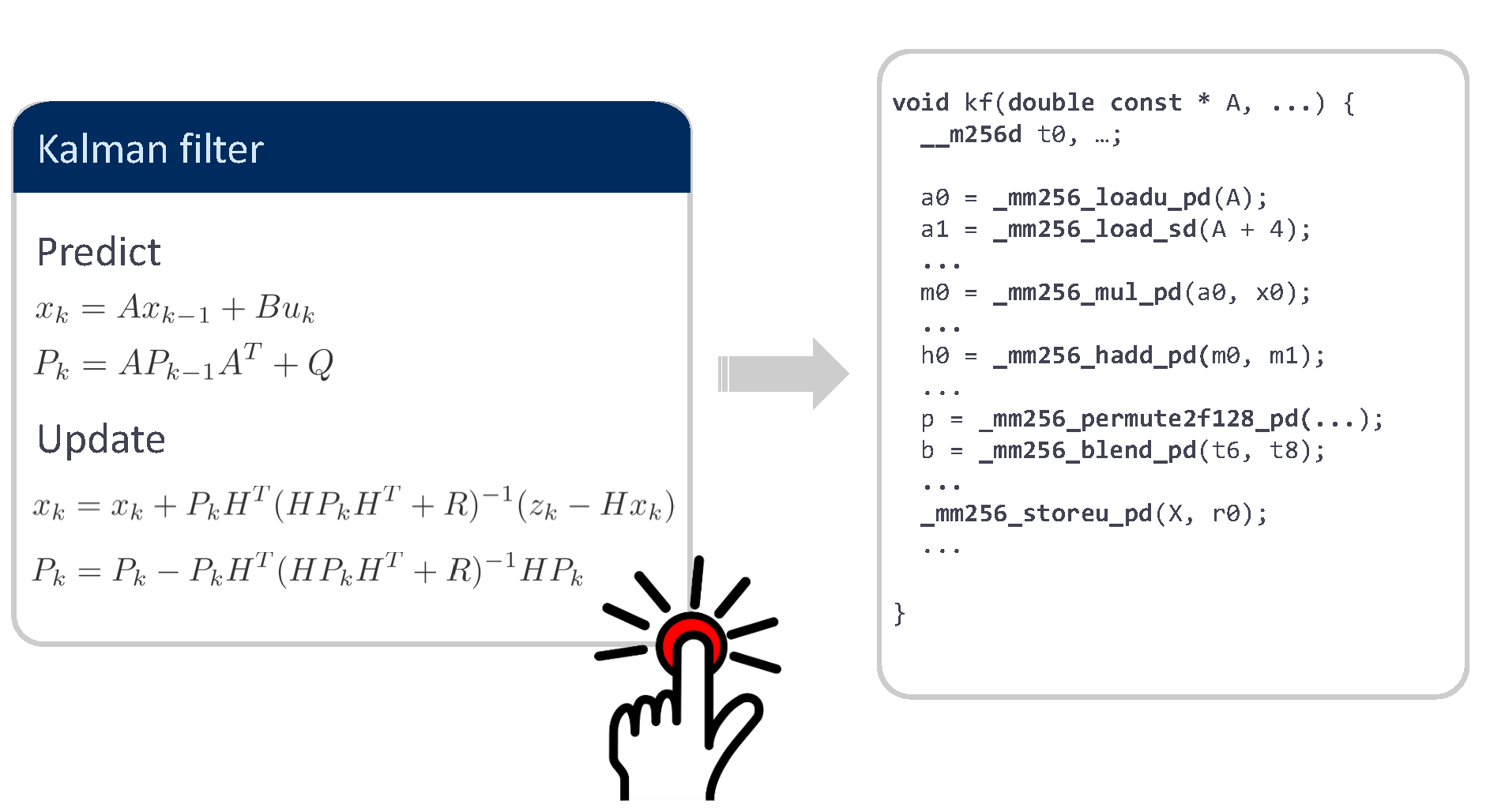## Explanation

We introduce SLinGen and LGen, program generators for small scale linear algebra computations. The input is a mathematical description (as in a book) of the algorithm. The output is optimized C code usning the available vector ISA. The project was executed in 3 steps with increasing functionality:

1. LGen: Basic linear algebra compiler: A compiler that translates basic operations (BLACs) on scalars, vectors, and matrices into vectorized C code [4,3].
2. LGen extension: Basic linear algebra compiler for structured matrices: Extensible framework inside LGen to support structured matrices .
3. SLinGen: Program generator for linear algebra: Building on 1. and 2. a generator for entire algorithms that are composed from linear algebra operations. This includes Cholesky factorization, solving Sylvester equations, and higher-level algorithms such as a Kalman filter .

We briefly explain the first step (LGen) below; see the publications below for more information.

By “basic linear algebra” we mean computations on matrices, vectors, and scalars that are composed from matrix multiplication, matrix addition, transposition, and scalar multiplication.

Examples of BLACs include:

• Simple computations, such as y = Ax.
• Computations that closely match the BLAS interface, e.g., $C = \alpha AB^T + \beta C$.
• Computations that need more than one BLAS call, e.g., $γ = x^T(A+B)y + \delta$.

The input to LGen is a BLAC including the (fixed) size of its operands. The output is an optimized C function, optionally using intrinsics for vectorization, that computes the BLAC.

## Code Generation Overview

The picture below provides an overview of LGen’s internal structure. LGen is designed after the version of Spiral that produces looped fixed size code.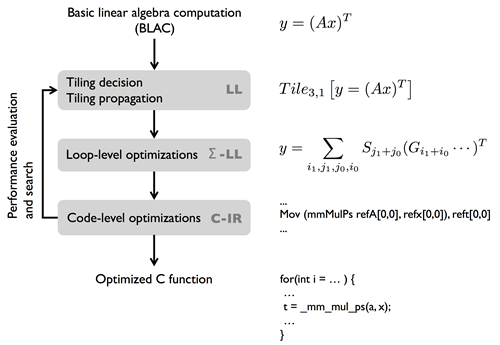A valid input to LGen is a BLAC with input and output operands of fixed size. The BLAC is specified in a DSL that we call Linear algebra Language (LL). In the first step, the tiling is fixed for the computation. The resulting fully-tiled computation is translated into a second DSL called Σ-LL, which is based on Σ-SPL used in Spiral. The latter is still a valid mathematical representation of the original BLAC but makes loops and access functions explicit. At this level LGen performs loop-level optimizations such as loop merging and exchange. Next, the Σ-LL expression is translated into a C intermediate representation (C-IR) for code-level optimizations, such as loop unrolling and translation into SSA form. Finally, the C-IR code is unparsed into C and performance results are used in the autotuning feedback loop.

Vectorization is done by decomposing the computation into a fixed set of nu-BLACs pre-implemented once for every vector architecture: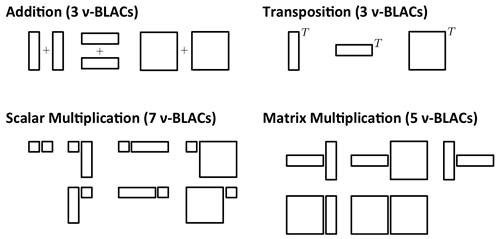More details about LGen and its vectorization process can be found in [4,3].

## Benchmarks

The plots below show performance results for four different classes of single precision floating point BLACs on an Intel Xeon X5680 with SSE 4.2 and 32 kB L1 D-cache. In the first three cases we use matrices with narrow rectangular shapes (panels) or small squares (blocks). The panel sizes are either n x 4 or 4 x n, chosen to fit into L1 D-cache. For the last case (micro-BLACs) the matrices are all n x n, with 2 ≤ n ≤ 10.

Case 1 (Simple BLAC): $y = Ax$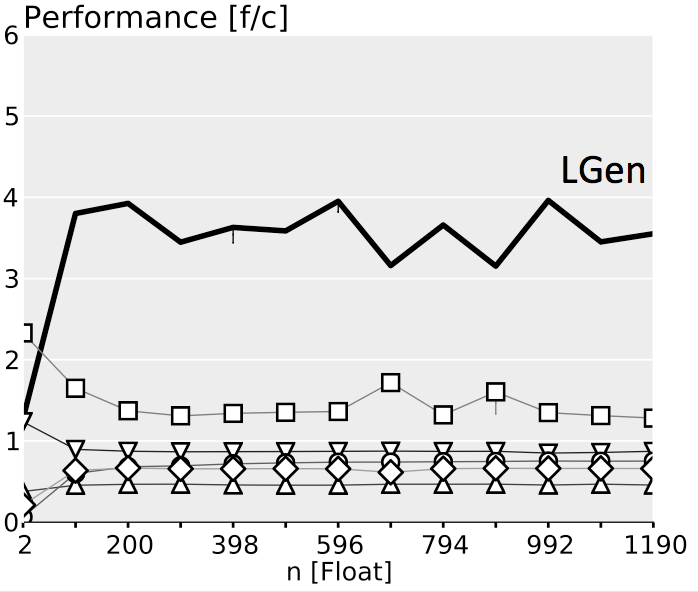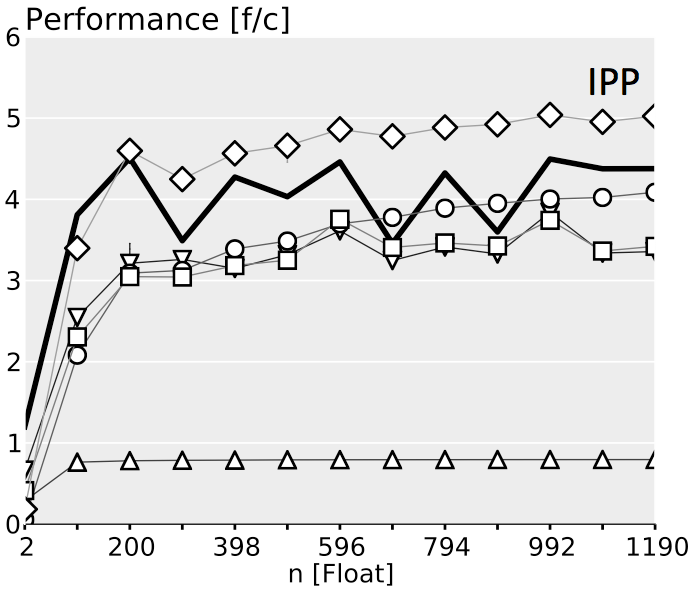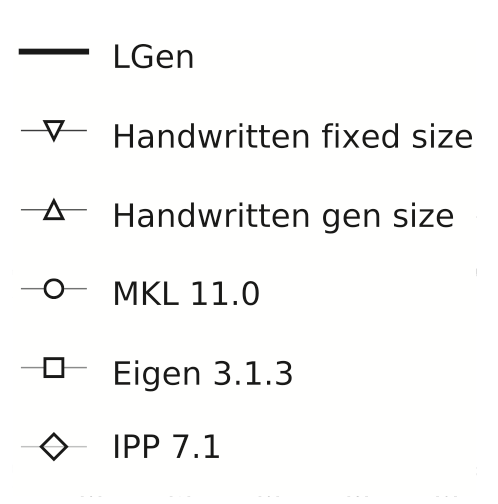$A\text{ is }n \times 4$ $A\text{ is }4 \times n$

Case 2 (BLAC that closely matches BLAS): $C = \alpha AB + \beta C$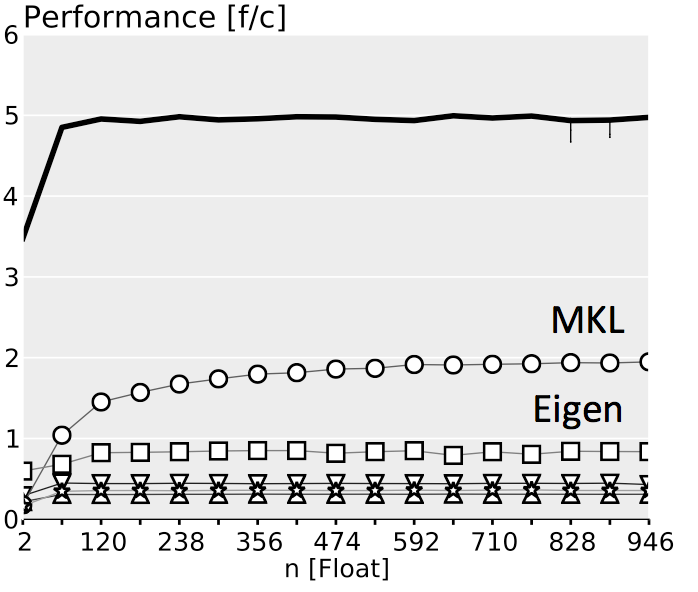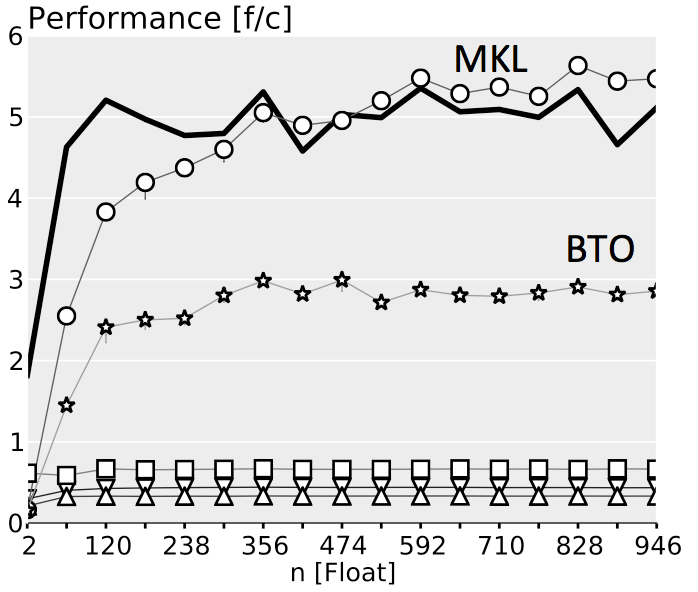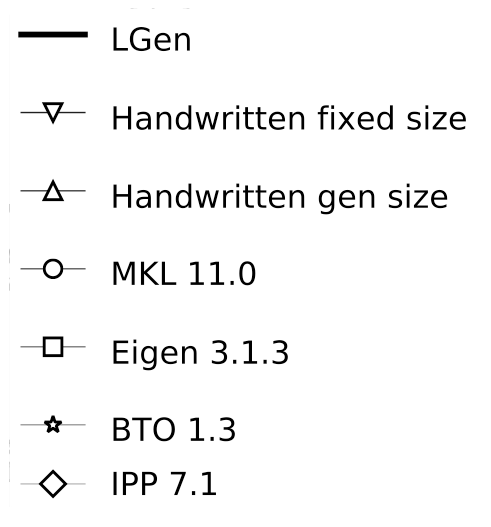$A\text{ is }n \times 4,\ B\text{ is }4 \times 4$ $A\text{ is }4 \times 4,\ B\text{ is }4 \times n$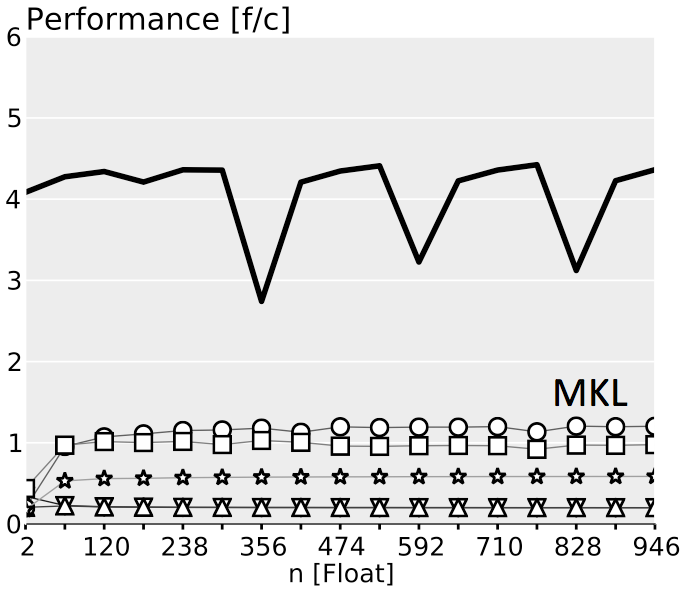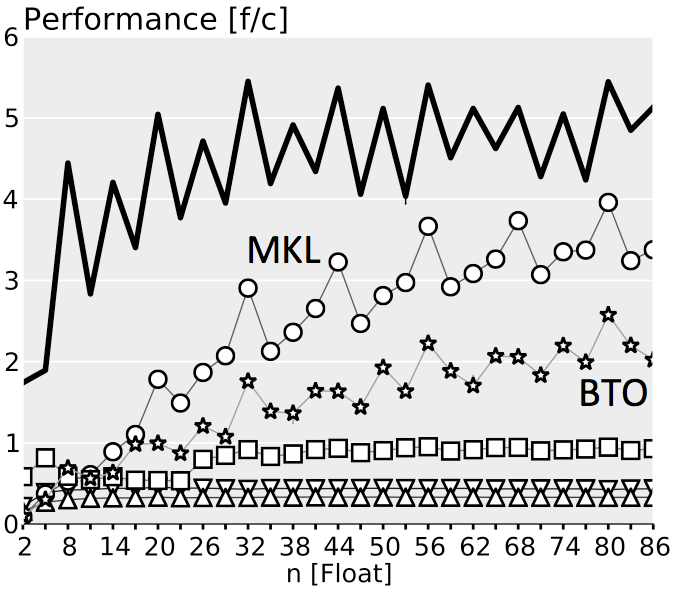$A\text{ is }4 \times n,\ B\text{ is }n \times 4$ $A\text{ is }n \times 4,\ B\text{ is }4 \times n$

Case 3 (BLAC that needs more than one BLAS call): $C = \alpha(A_0 + A_1)^T B + \beta C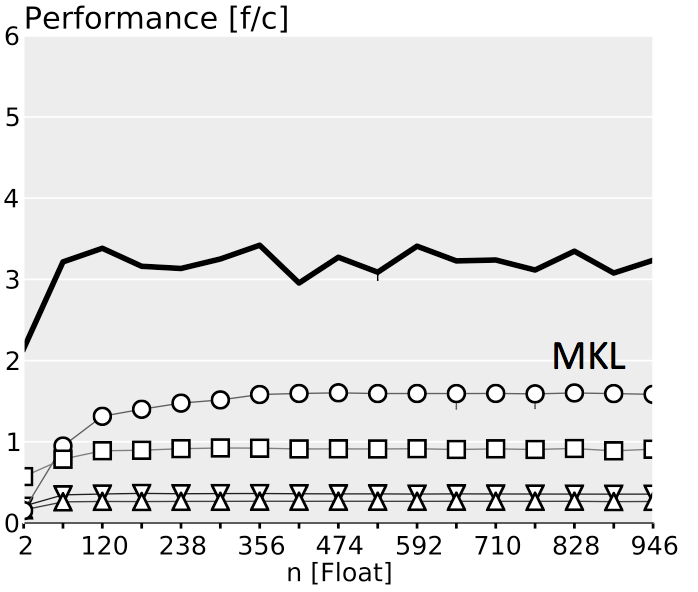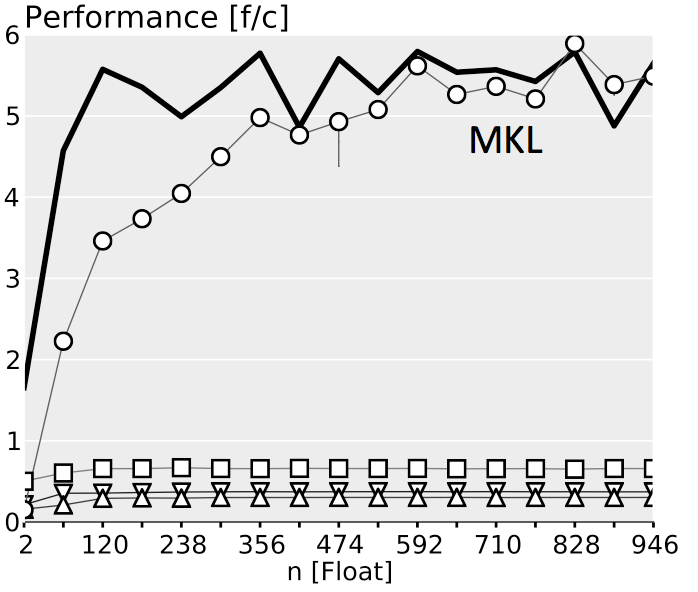$A\text{ is }4\times n, B\text{ is }4 \times 4A_0\text{ is }4 \times 4,\ B\text{ is }4 \times n$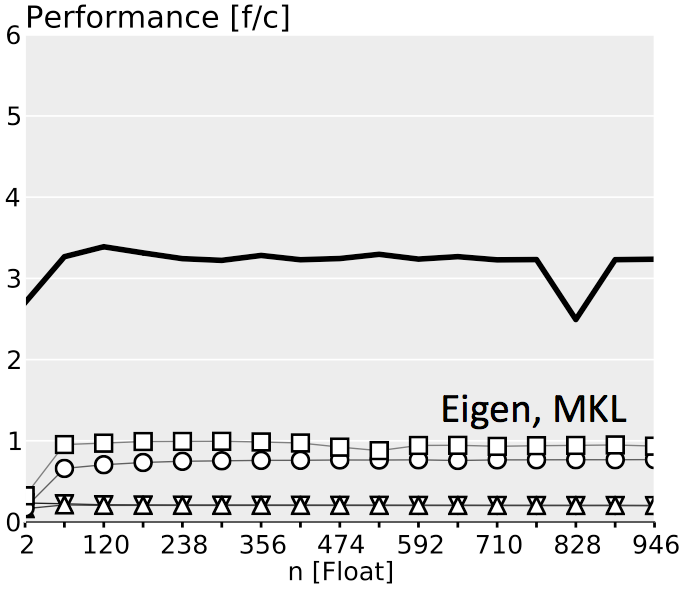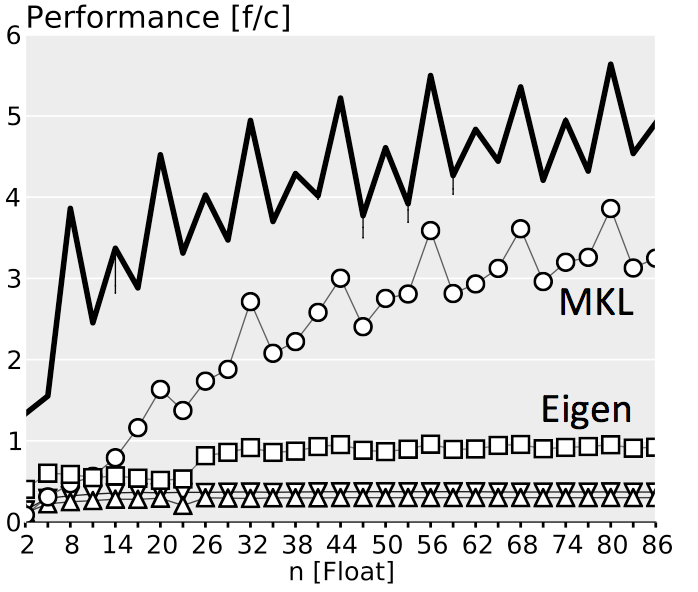$A_0\text{ is }n \times 4,\ B\text{ is }n \times 4A_0\text{ is }4 \times n,\ B\text{ is }4 \times n$Case 4 (Micro-BLACs): Sizes$2 \leq n \leq 10$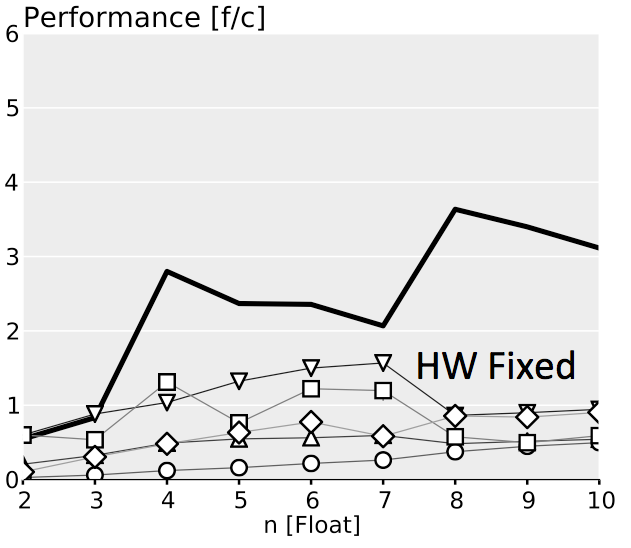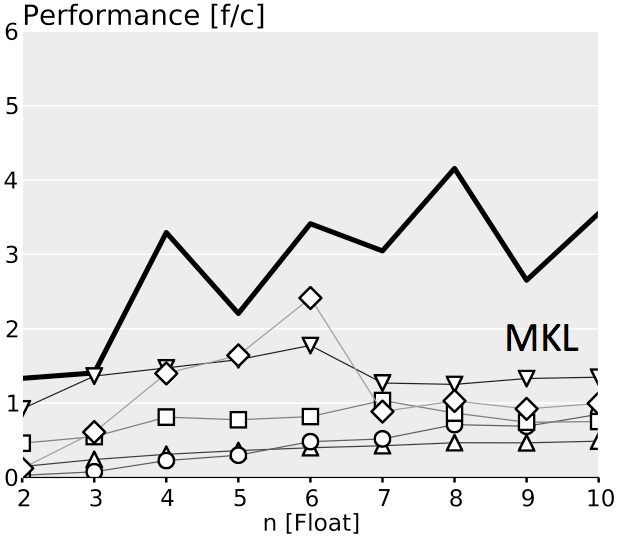$y = AxC = AB$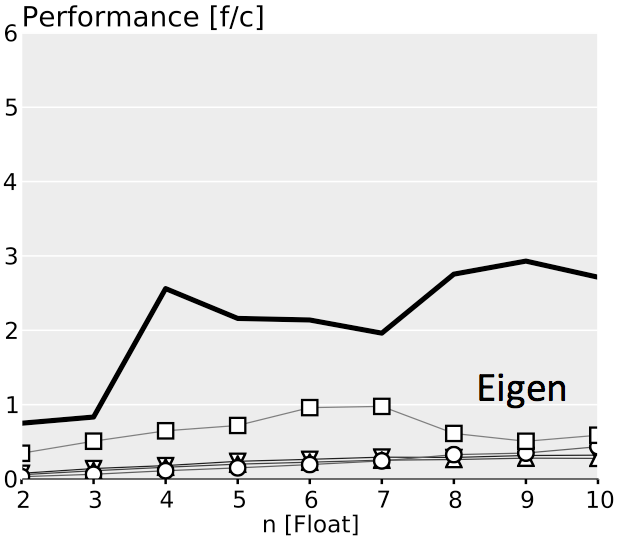$\delta = x^T Ay\$

## Source

SLinGen (which contains LGen) is available at danielesgit/slingen.

## Contact

Daniele Spampinato (spampinato at cmu.edu)

## Main References

1. Daniele Spampinato, Diego Fabregat-Traver, Paolo Bientinesi, Markus Püschel
Program Generation for Small-Scale Linear Algebra Applications
Proc. Code Generation and Optimization (CGO), pp. 327–339, 2018

2. Daniele G. Spampinato and Markus Püschel
A Basic Linear Algebra Compiler for Structured Matrices
Proc. International Symposium on Code Generation and Optimization (CGO), pp. 117-127, 2016
CGO 2016 highest ranked artifact

3. Nikolaos Kyrtatas, Daniele G. Spampinato and Markus Püschel
A Basic Linear Algebra Compiler for Embedded Processors
Proc. Design, Automation and Test in Europe (DATE), pp. 1054-1059, 2015

4. Daniele G. Spampinato and Markus Püschel
A Basic Linear Algebra Compiler
Proc. International Symposium on Code Generation and Optimization (CGO), pp. 23-32, 2014